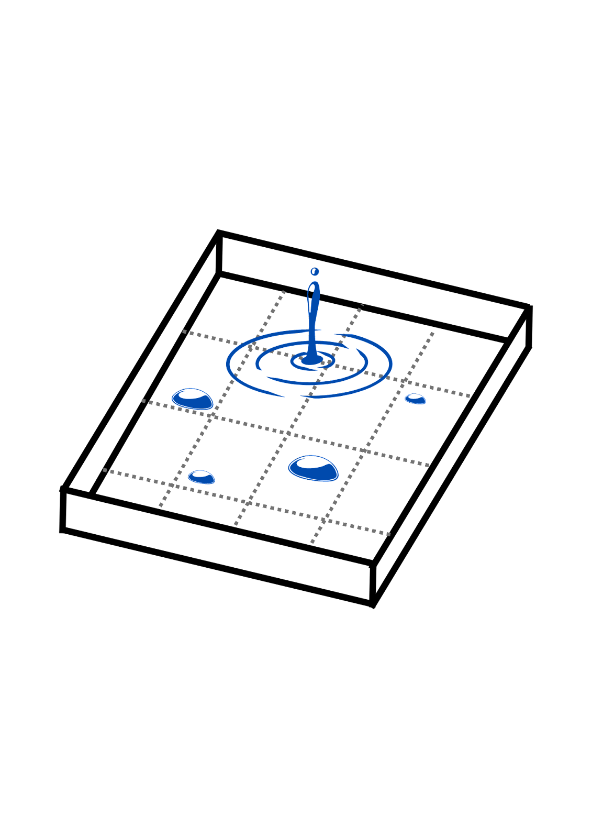Categories

# February 2023 – Another way to see the synchronization

Author: Andrea Arlette ESPAÑA-TINAJERO

Universidad Autónoma de San Luis Potosí, México

Aix-Marseille Université, France

When talking about the synchronization phenomenon, the most common thing is to think of biological events where it is possible to observe it with our own eyes, for example, when dozens of birds fly in the sky and form various patterns with choreographies that seem very well rehearsed. Also, some types of fish exhibit this type of behavior when swimming; it seems that they do it harmoniously and without colliding with each other .

In our case, we will think of the synchronization phenomenon in the following way: it will be a process in which a set of agents interacts, and by allowing a long enough period to pass, then all of them will have the same state, which we will call synchronized state. In the bird example, the synchronized state might be that they are all flying north, and in the fish example, that they are all swimming south.

With this concept of synchronization that has just been defined, we can now think of another type of phenomenon, in particular, the following: consider an empty, impermeable box, without a lid (to be able to observe what happens inside), in which we will pour a liquid (none in particular, we can think of water or oil), with a volume large enough to cover the entire surface of the box. Intuition tells us that when we finish pouring the liquid, each unit of surface area of the box will be the basis of the same volume of liquid. This would be the synchronized state, and this process is more commonly called diffusion.Figure One: Impermeable box, with markings on each area unit. Drops of different sizes and the place where the liquid is poured are shown, as well as the circular neighbourhood formed by pouring the liquid into the box.

The way in which the liquid fills the box is not a very surprising mechanism; that is, if the liquid begins to pour at a point, then a circular neighborhood around that point is expected to fill uniformly at the same time, until the entire area of the box is covered, and then, we will see how its volume increases, until the liquid runs out.

On the other hand, what happens if the box is not initially empty? Suppose that on the surface of the box there are drops of different volumes, and the liquid begins to pour at a point where there is no drop. Diffusion occurs as in the case of the empty box, but when the liquid interacts with a drop, then the circular neighborhood changes its shape to become a kind of deformed number 8, and so on with the drops that it finds in its path. What happens is a kind of agglutination phenomenon, that is, how one drop sticks to another, just before the area of the box fills up and begins to increase in volume until the liquid runs out.

So, for the case where there are drops, how could we describe the way the box is filled? First, we can identify that this phenomenon depends on some factors, the first, how many drops there are in the box at the beginning and what volume each of them has. You can even think of the effect if the box is not square or has holes inside it (without considering liquid losses, each of the holes would have a barrier that would prevent liquid loss).

Despite these new obstacles, the ways in which the synchronized state is reached are accurately described using mathematics, thanks to a new combinatorial approach. In this approach, the configurations of the droplets are encoded together with their volume and the total volume of the liquid. In this way, its route towards synchronization is fully determined .

The kind of mathematical objects that are used to describe and code the paths to synchronization are very simple to understand. They are called discrete increasing functions that go above the diagonal and below the constant. They take a list of length N, considering that in each place a number greater than or equal to the one on the left and less than the one on the right is placed. For example, when N=5, the diagonal is (1,2,3,4,5), the constant is (5,5,5,5,5) and two functions between them would be (2,2,3,4,5) and (2,2,4,4,5). These mathematical objects have been extensively studied; multiple characteristics and properties of them are known.

In physics, knowing how to use the tools provided by mathematics, which usually focus on calculus, differential equations, probability, and statistics, have allowed us to solve many problems in an elegant, useful, and educational way. In this case, using combinatorial and number theory tools allowed us to make an exact description of what happens before reaching synchronization, which in turn is a widely observed and studied phenomenon. No tool is left over, one day it could help us to graduate with a doctorate.

References:

1. A. Pikovsky, M. Rosenblum, J. Kurths, Synchronization – a universal concept in nonlinear sciences, in: Cambridge Nonlinear Science Series, 2001.
2. A. España, X. Leoncini, E. Ugalde, Combinatorics of the paths towards synchronization, 2022. doi:10.48550/ARXIV.2205.05948. URL: https://arxiv.org/abs/2205.05948